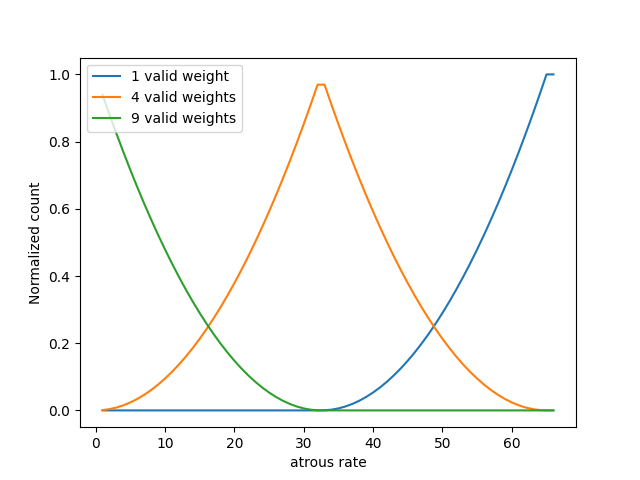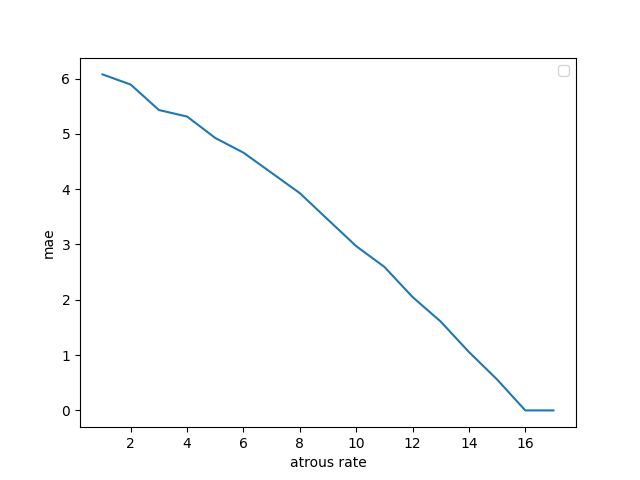# 膨胀卷积与 1x1 卷积

ASPP with different atrous rates effectively captures multi-scale information. However, we discover that as the sampling rate becomes larger, the number of valid filter weights (i.e., the weights that are applied to the valid feature region, instead of padded zeros) becomes smaller. In the extreme case where the rate value is close to the feature map size, the 3 × 3 filter, instead of capturing the whole image context, degenerates to a simple 1 × 1 filter since only the center filter weight is effective.

import torch
import numpy as np
import matplotlib.pyplot as plt

if __name__ == '__main__':
batch_size, num_channels, height, width = 1, 1, 65, 65
x = torch.ones(batch_size, num_channels, height, width)

weight = torch.ones(1, 1, 3, 3)
num_valid_weights_1 = []
num_valid_weights_4 = []
num_valid_weights_9 = []
dilations = range(1, height + 2)
for dilation in dilations:
y = torch.nn.functional.conv2d(x, weight, bias=None, stride=1,
dilation=[dilation, dilation])
num_valid_weights_1.append(len(torch.nonzero(y == 1)))
num_valid_weights_4.append(len(torch.nonzero(y == 4)))
num_valid_weights_9.append(len(torch.nonzero(y == 9)))
num_valid_weights_1 = np.asarray(num_valid_weights_1) / np.prod(x.shape)
num_valid_weights_4 = np.asarray(num_valid_weights_4) / np.prod(x.shape)
num_valid_weights_9 = np.asarray(num_valid_weights_9) / np.prod(x.shape)
plt.plot(dilations, num_valid_weights_1, label='1 valid weight')
plt.plot(dilations, num_valid_weights_4, label='4 valid weights')
plt.plot(dilations, num_valid_weights_9, label='9 valid weights')
plt.xlabel('atrous rate')
plt.ylabel('Normalized count')
plt.legend(loc='best')
plt.show()import torch
import matplotlib.pyplot as plt

if __name__ == '__main__':
batch_size, num_channels, height, width = 2, 8, 16, 16
x = torch.randn(batch_size, num_channels, height, width)

weight = torch.randn(4, 8, 3, 3)
weight_1x1 = weight[:, :, 1:2, 1:2]
y_1x1 = torch.nn.functional.conv2d(x, weight_1x1, bias=None, stride=1)
dilations = range(1, height + 2)
mae_list = []
for dilation in range(1, height + 2):
y = torch.nn.functional.conv2d(x, weight, bias=None, stride=1,
dilation=[dilation, dilation])
mae_list.append(torch.mean(torch.abs(y - y_1x1)))
plt.plot(dilations, mae_list)
plt.xlabel('atrous rate')
plt.ylabel('mae')
plt.legend(loc='best')
plt.show()• 20210723, 发布